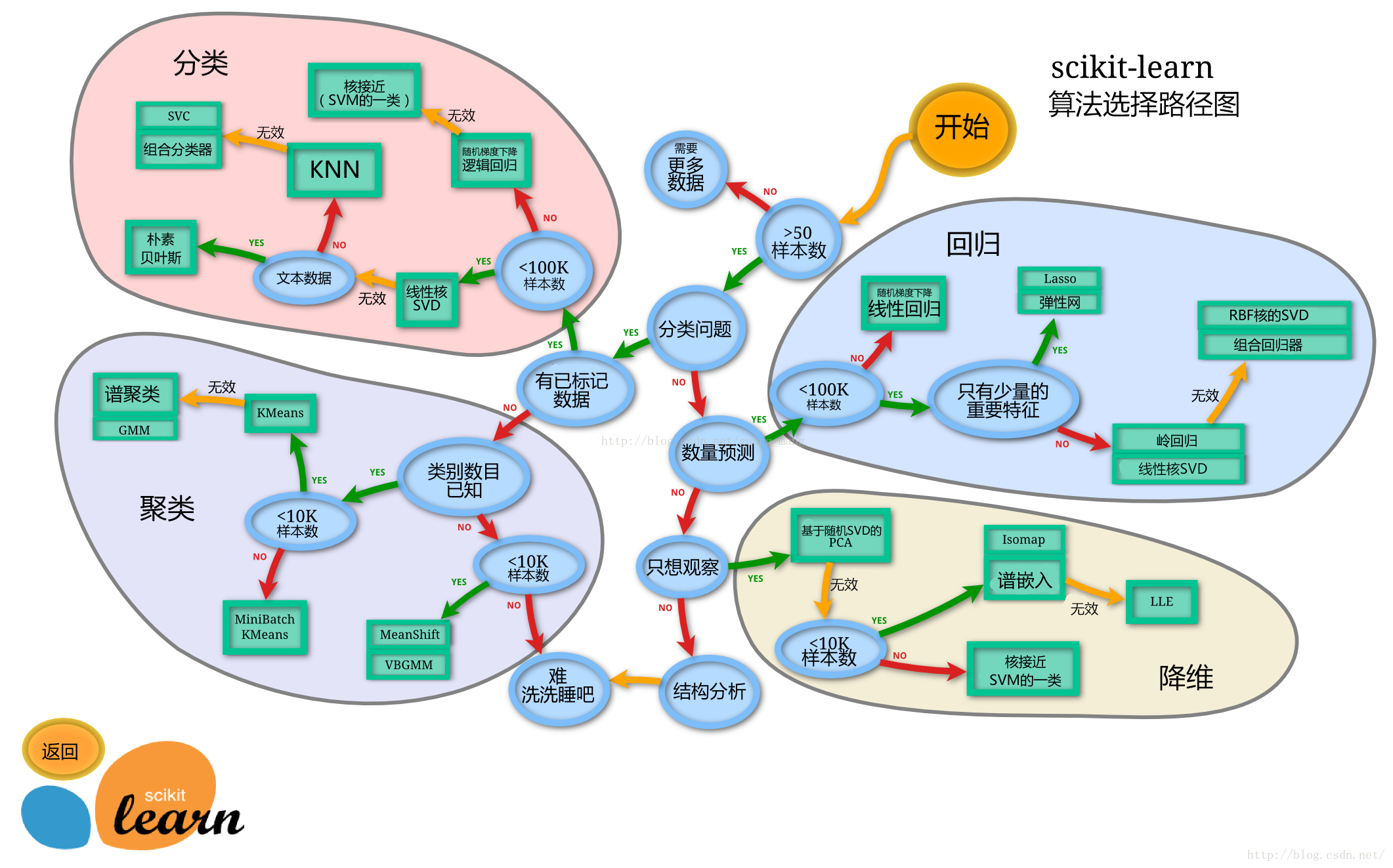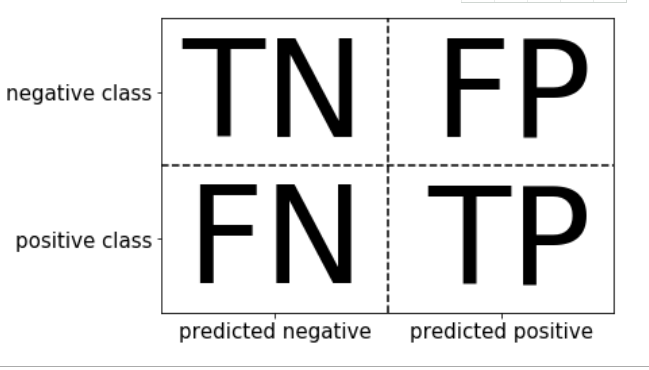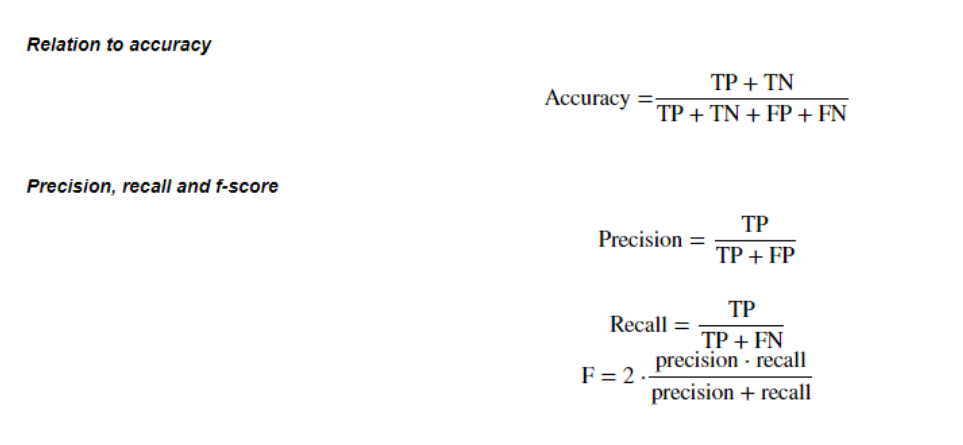动手学数据分析（5）模型建立与评估

• 了解数据建模
• 使用sklearn完成分类模型的构建

• 了解模型评估
• 使用sklearn完成模型评估

数据建模

• 任务目标：指出数据中隐藏的模式。
之所以称为无监督学习，是因为我们并不知道要找的模式是什么，而是要依靠算法从数据集中发现模式。
什么是监督学习？
• 任务目标：使用数据中的模式做预测
他们的预测都基于已有的模式

• 任务目标：使用数据中的模式做预测，并根据越来越多的反馈结果不断改进。
无监督学习和监督学习模型在部署之后便无法更改，不同于此，强化学习模型自身可以通过反馈结果不断改进。

# sklearn模型算法选择路径图
Image('sklearn.png')1. 切割数据集
在开始建模之前，还需要把数据集拆分为两个部分：训练集和测试集。在普通机器学习项目中至少需要包括这两个数据集，一个用于训练机器，确定模型。另一个用于测试模型的准确性。不仅如此还需要一个验证集，以在最终测试之前增加验证环节

【思考】划分数据集的方法有哪些？

from sklearn.model_selection import train_test_split
# 一般先取出X和y后再切割，有些情况会使用到未切割的，这时候X和y就可以用,x是清洗好的数据，y是我们要预测的存活数据'Survived'
X = data
y = train['Survived']
# 对数据集进行切割
X_train, X_test, y_train, y_test = train_test_split(X, y, stratify=y, random_state=0)
# 查看数据形状
X_train.shape, X_test.shape

sklearn中的train_test_split函数，是机器学习中拆分数据集的常用工具。

stratify依据标签y，按原数据y中各类比例，分配给train和test，使得train和test中各类数据的比例与原数据集一样
random_state用于数据集拆分过程的随机化设定。如果指定了一个整数，那么这个数叫做随机化种子，每次设定固定的种子能够保证得到同样的训练集和测试集，否则进行随机分割
train_data所要划分的样本特征集
train_target所要划分的样本结果
test_size样本占比,可以为浮点、整数或None，默认为None，若为浮点时，表示测试集占总样本的百分比，若为整数时，表示测试样本样本数，若为None时，test size自动设置成0.25
train_size可以为浮点、整数或None，默认为None，若为浮点时，表示训练集占总样本的百分比，若为整数时，表示训练样本的样本数，若为None时，train_size自动被设置成0.75

【思考】什么情况下切割数据集的时候不用进行随机选取？

1. 建立模型
创建基于线性模型的分类模型
最常见的两种线性分类算法是Logistic回归（logistic regression）和线性支持向量机（linear support vector machine,线性SVM）

from sklearn.linear_model import LogisticRegression
from sklearn.svm import LinearSVC

X,y=mglearn.datasets.make_forge()

fig,axes=plt.subplots(1,2,figsize=(10,3))

for model,ax in zip([LinearSVC(),LogisticRegression()],axes):
clf=model.fit(X,y)
mglearn.plots.plot_2d_separator(clf,X,fill=False,eps=0.5,ax=ax,alpha=.7)

mglearn.discrete_scatter(X[:,0],X[:,1],y,ax=ax)
ax.set_title("{}".format(clf.__class__.__name__))
ax.set_xlabel("Feature 0")
ax.set_ylabel("Feature 1")
axes.legend()

print("Training set score: {:.2f}".format(lr.score(X_train, y_train)))
print("Testing set score: {:.2f}".format(lr.score(X_test, y_test)))

from sklearn.model_selection import train_test_split  #从sklearn中导入适当的评估器类，选择模型类
Xtrain,Xtest,Ytrain,Ytest =train_test_split(wine.data,wine.target,test_size=0.3)  #实例化
clf=DecisionTreeClassifier(random_state=0)
rfc=RandomForestClassifier(random_state=0)
clf=clf.fit(Xtrain,Ytrain)    #调用模型的fit方法对数据进行拟合
rfc=rfc.fit(Xtrain,Ytrain)
score_c=clf.score(Xtest,Ytest)   #评估模型
score_r=rfc.score(Xtest,Ytest)
print("Single Tree:{}".format(score_c)
,"Random Forest:{}".format(score_r))

max_features默认为auto。“auto”/“sqrt”（总特征个数开平方取整）,“log2”（总特征个数取对数取整）这个参数在决策树中也有其默认为None，即有多少特征用多少特征。
n_estimators决策树个数，默认100。
bootstrap每次构建树是否采用有放回样本的方式抽取数据集，默认为True。
oob_score是否使用袋外数据来评估模型，默认为False。
boostrap和 oob_score这两个参数一般要配合使用。如果boostrap是False，那么每次训练时都用整个数据集训练，如果boostrap是True，那么就会产生袋外数据。
max_samples构建每棵树需要抽取的最大样本数据量，默认为None
n_jobs:设定fit和predict阶段并列执行的CPU核数,如果设置为-1表示并行执行的任务数等于计算机核数。默认为None，即采用单核计算。
verbose控制构建数过程的冗长度，默认为0。
warm_start当设置为True,重新使用之前的结构去拟合样例并且加入更多的估计器(estimators,在这里就是随机树)到组合器中。默认为 False

① 基于泛化误差与模型复杂度的关系来进行调参；

② 根据对模型的影响程度，由大到小对参数排序，并确定哪些参数会使模型复杂度减小，哪些会增大；

③ 依次选择合适的参数，通过绘制学习曲线或网格搜索的方法调参，直到找到最大准确得分。

【思考】为什么线性模型可以进行分类任务，背后是怎么的数学关系？

【思考】对于多分类问题，线性模型是怎么进行分类的？

1. 输出模预测结果
一般监督模型在sklearn里面有个predict能输出预测标签，predict_proba则可以输出标签概率
# 预测标签
pred = lr.predict(X_train)
# 此时我们可以看到0和1的数组
pred[:10]
# 预测标签概率
pred_proba = lr.predict_proba(X_train)
pred_proba[:10]

模型评估

• 正利率：被模型正确预测为正类别的样本所占的比例。
正利率=正例数/（正例数+假负例数）

• 假正利率：被模型错误预测为正类别的样本所占的比例。
假正利率=假正例数/（假正例数+负例数）

from sklearn.metrics import roc_curve
#ROC曲线在sklearn中的模块为sklearn.metrics
fpr, tpr, thresholds = roc_curve(y_test, lr.decision_function(X_test))
plt.plot(fpr, tpr, label="ROC Curve")
plt.xlabel("FPR")
plt.ylabel("TPR (recall)")
# 找到最接近于0的阈值
close_zero = np.argmin(np.abs(thresholds))
plt.plot(fpr[close_zero], tpr[close_zero], 'o', markersize=10, label="threshold zero", fillstyle="none", c='k', mew=2)
plt.legend(loc=4)

Image('Snipaste_2020-01-05_16-37-56.png')

from sklearn.model_selection import cross_val_score

k折交叉验证分数

lr = LogisticRegression(C=100)
scores = cross_val_score(lr, X_train, y_train, cv=10)

print("Average cross-validation score: {:.2f}".format(scores.mean()))from sklearn.metrics import confusion_matrix
# 训练模型
lr = LogisticRegression(C=100)
lr.fit(X_train, y_train)
pred = lr.predict(X_train)
# 混淆矩阵
confusion_matrix(y_train, pred)
from sklearn.metrics import classification_report
# 精确率、召回率以及f1-score
print(classification_report(y_train, pred))

• 一般情况下，都会用：K折交叉验证来分离数据集。
• 数据量比较大，或者算法效率较低的情况下会考虑：分离训练数据集和评估数据
• 平衡评估算法，模型训练的速度以及数据集的大小:弃一交叉验证和重复随机评估、训练集分离

热门文章jira安装配置指南（Ubuntu16.04）内含解决connection refused问题方法那些年让我们目瞪口呆的bugredis的下载与安装《大话数据结构》第三章学习笔记--线性表（一）对象的扩展让程序员最头疼的5种编程语言写论文注意事项安卓 串口开发2021-2027年中国铪市场调研与发展趋势分析报告

2021-2027年中国铪市场调研与发展趋势分析报告 本报告研究中国市场铪的生产、消费及进出口情况&#xff0c;重点关注在中国市场扮演重要角色的全球及本土铪生产商&#xff0c;呈现这些厂商在中国市场的铪销量、收入、价格、毛利率、市场份额等关键指标。此外&#xff0c;针对…Aggressive cows题目翻译剖析组建PMO的6个大坑︱PMO深度实践Flowable入门系列文章118 - 进程实例 07

1、获取流程实例的变量 GET运行时/进程实例/ {processInstanceId} /变量/ {变量名} 表1.获取流程实例的变量 - URL参数 参数需要值描述processInstanceId是串将流程实例的id添加到变量中。变量名是串要获取的变量的名称。 表2.获取流程实例的变量 - 响应代码 响应码描述200指…微信每天自动给女[男]朋友发早安和土味情话C语言二分查找详解【普通人VS程序员】电脑还可以这样关机，神操作，学到了学到了~（收藏起来慢慢学）项目经理，你有什么优势吗？nginx的负载均衡和故障转移

#注&#xff1a;proxy_temp_path和proxy_cache_path指定的路径必须在同一分区 proxy_temp_path /data0/proxy_temp_dir; #设置Web缓存区名称为cache_one&#xff0c;内存缓存空间大小为200MB&#xff0c;1天没有被访问的内容自动清除&#xff0c;硬盘缓存空间大小为30GB。 pro…业务逻辑漏洞